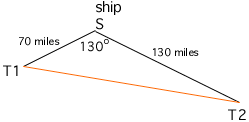A ship at sea is 70 miles from one transmitter and 130 miles from another. The measurement of the angle between the signals is 130 degrees. How far apart are the transmitters? Jeff Hi Jeff, In the diagram below T1 is the position of the first transmitter and T2 is the position of the second transmitter.The Law of Sines and the Law of Cosines are useful tools for problem of this type, one where you need to find a side length or an angle in a triangle. A statement of each of these Laws is in the answer to a previous question titled The angles in a triangle. In your problem you know the lengths of two sides and the measure of the angle between them, and what you want is the length of the third side. This is exactly the information you can find from the Law of Cosines. Using the notation in the reference mentioned above, b = 70 miles, c = 130 miles and A = 130 o. Cheers, Penny Go to Math Central